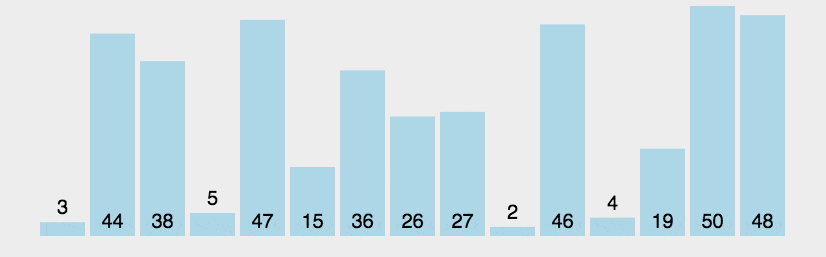# JavaScript冒泡排序+Vue可视化冒泡动画

2022-12-26 19:51:20  阅读：107  来源： 互联网

# 01、JavaScript冒泡排序

## 1.1、原理

• 、从第一位元素100（0索引）开始，比较相邻`arr、arr`元素的大小，大的排后面，如果`arr>arr`则交换值位置。
• 、依次相邻元素比较、交换，一轮完成后，最大元素就到了最右边了。这个过程中，最大的元素（最大的泡泡）就像冒泡一样到了末尾。
• ③、然后继续对剩下的前面`len-1=5`个元素重复上述步骤，直到只剩下一个元素。这是一个递归的过程，递归到第一个元素，就完成了冒泡排序。## 1.2、JavaScript实现

```
```
 `//经典冒泡排序算法` `//从小到大冒泡排序` `let arr = [100, 5, 6, 17, 3, 1];` `let count=0; //计数器` `function bubbleSort(arr) {` `const len = arr.length;` `let t;count=0;` `for (let i = 0; i < len - 1; i++) {` `for (let j = 0; j < len - i - 1; j++) {` `count++;` `//比较相邻两个元素` `if (arr[j] > arr[j + 1]) {` `//交换两个元素，大的往后排列` `t = arr[j];` `arr[j] = arr[j + 1];` `arr[j + 1] = t;` `}` `}` `}` `return arr;` `}` `console.log(bubbleSort(arr),"比较次数：",count);` `//[1, 3, 5, 6, 17, 100] '比较次数：' 15`

```
```
 `let arr = [100, 5, 6, 17, 3, 1];` `function bubbleSort(arr) {` `const len = arr.length;` `for (let i = 0; i < len - 1; i++) {` `for (let j = 0; j < len - i - 1; j++) {` `//比较相邻两个元素` `if (arr[j] > arr[j + 1]) {` `//用结构赋值进行交换` `[arr[j], arr[j + 1]] = [arr[j+1], arr[j]];` `}` `}` `}` `return arr;` `}` `console.log(bubbleSort(arr));` `//[1, 3, 5, 6, 17, 100]`

# 02、Vue实现一个冒泡动画

## 2.1、排序过程改造

• 定义一个排序对象`SortItem`，包装待排序元素，用于可视化展示，属性包括排序值、泡泡大小、泡泡颜色。
• 用上面的排序对象`SortItem`，生成排序对象集合，正式排序步骤中用该集合。方法的参数为排序元素字符串，空格隔开，如“9 100 6 17 3 1”。

2. 关于本站的所有留言、评论、转载及引用，纯属内容发起人的个人观点，与本站观点和立场无关；
3. 关于本站的所有言论和文字，纯属内容发起人的个人观点，与本站观点和立场无关；
4. 本站文章均是网友提供，不完全保证技术分享内容的完整性、准确性、时效性、风险性和版权归属；如您发现该文章侵犯了您的权益，可联系我们第一时间进行删除；
5. 本站为非盈利性的个人网站，所有内容不会用来进行牟利，也不会利用任何形式的广告来间接获益，纯粹是为了广大技术爱好者提供技术内容和技术思想的分享性交流网站。